EXERCISE 5.6

1) Name the types of following triangles :

(a) Triangle with length of sides 7 cm, 8 cm and 9 cm.
Ans : Since  the lengths of the three sides are different it will be a scalene  triangle.

(b) ∆ ABC with AB = 8.7 cm, AC = 7 cm and BC = 6 cm.
Ans : Since  the lengths of the three sides are different it will be a scalene  triangle.

(c) ∆ PQR , such that PQ = QR = PR = 5 cm.
Ans : Since  the lengths of the three sides are equal it will be an equilateral triangle.

(d) ∆ DEF, with m∠D = m 90˚
Ans : Since one angle is 90º , it will be a right angled triangle.

(e) ∆XYZ, with m ∠ Y = 90˚
Ans : Since one angle is 90º , it will be a right angled triangle.

(f) ∆ LMN with m ∠L = 30˚, m ∠ M = 70˚ and m ∠ N = 80˚.
Ans : Since  the largest angle is less than 90º so it will be an acute  angled triangle.

2) Match the following:

 Measures of Triangle Types of Triangle (1) 3 sides of equal length (a) Scalene (2) 2 sides of equal length (b) Isosceles right angled (3) All the sides are of different length (c) Obtuse angled (4) 3 acute angles (d) Right angled (5) 1 right angle (e) Equilateral (6) 1 Obtuse angle (f) Acute – angled (7) 1 right angle with two side of equal length (g) Isosceles

Ans : (1) → (e) ,      (2) → (g) ,      (3) → (a) ,      (4) → (f) ,              (5) → (d) ,    (6) → (c),          (7) → (b).

3) Name each of the following triangles in two different ways : (you may judge the nature of the angle by observation)
a)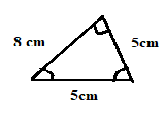b)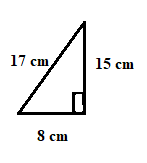c)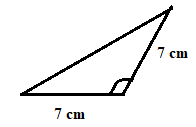d)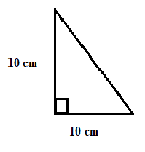e)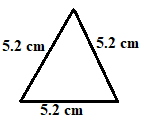f)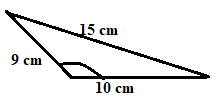Ans : (a) Acute angled triangle and Isosceles triangle.
(b) Right angled triangle and Scalene triangle.
(c) Obtuse  angled triangle and Isosceles triangle.
(d) Right  angled triangle and Isosceles.
(e) Acute angled triangle and Equilateral triangle..
(f) Obtuse angled triangle and Scalene triangle.

4) Try to construct triangles using match sticks some are shown here.
Can you make a triangle with.
(a) 3 matchsticks?            (b) 4 matchsticks?
(c) 5 matchsticks?            (d) 6 matchsticks?
(Remember you have to use all the given matchsticks in each case)
Name the type of triangle in each case. If you cannot make a triangle, think of reasons for it.
Ans :
(a) Yes, with 3 match sticks
We can make an equilateral triangle

(b) Not possible. We know that sum of the length of any two sides of a triangle is always greater than the third side and hence we cannot make a triangle with 4 matchsticks.
(c) Yes,
The above triangle is an isosceles triangle.

(d) Yes,
We can make an equilateral triangle with
matchsticks.